# 寫程式的基本功-排序演算法(Sorting Algorithm)

## 快速排序法(Quick sort)

### 圖解快速排序法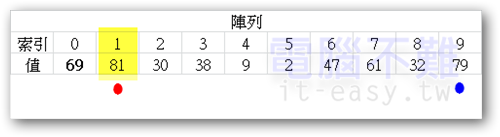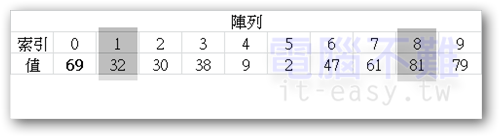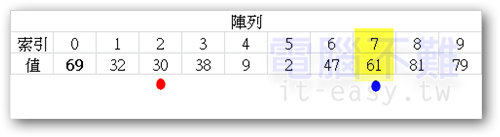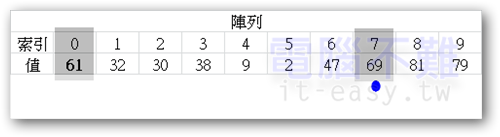pivot改變後，繼續搜尋。很快的藍點又找到了比pivot小的數。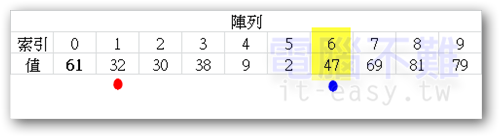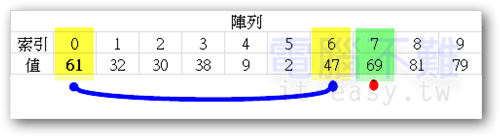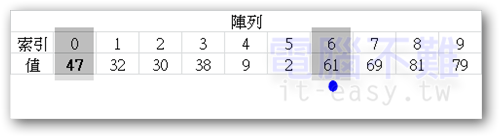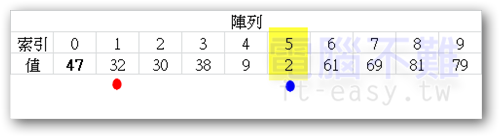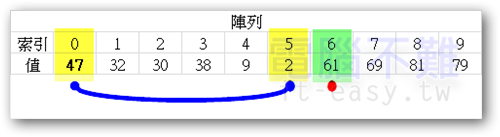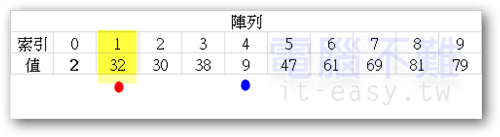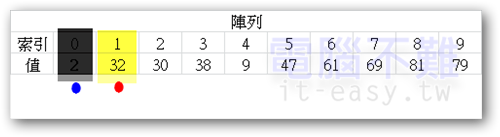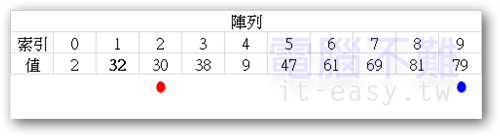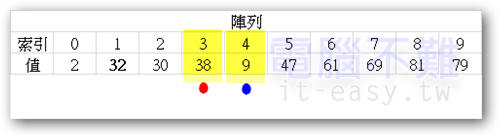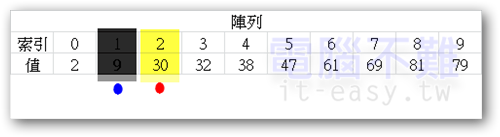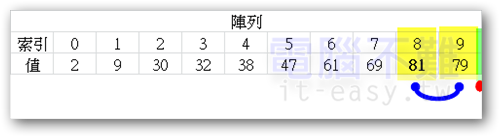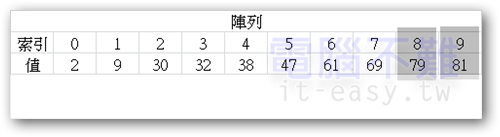### 快速排序法程式碼

``` public static int[] exchange_sort(int[] ori_arr, boolean isIncrease){ //交換排序（Exchange sort） int[] arr=ori_arr.clone(); //將arr陣列位址指向新複製出來的ori_arr陣列。確保原始陣列資料不會改變。 int len=arr.length; //取得陣列長度 for(int i=0;i<len-1;i++){ for(int k=i+1;k<len;k++){ if((isIncrease&&arr[i]>arr[k])||(!isIncrease&&arr[i]<arr[k])){ //遞增遞減判斷 int buffer=arr[i]; arr[i]=arr[k]; arr[k]=buffer; } } } return arr; } ```